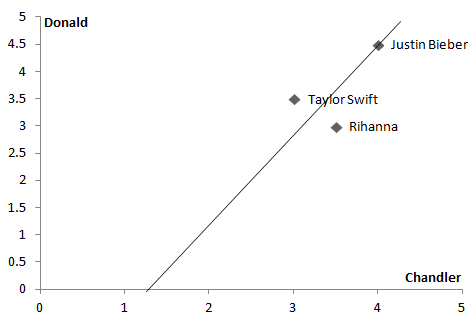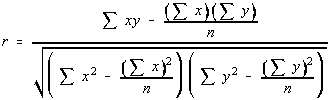# Finding similarities using Pearson Correlation Score

 Category: Machine Learning Tags:

## Introduction

In our previous article, we learned about Euclidean Distance Score and we have seen how we can use score to find similarities. In this article we are going to learn about a different mathematical formula which will also give us a score usually called correlation coefficient.

The correlation coefficient is about drawing a best fit straight line between two sets of data. If line pass by data points it means two sets are most similar, if data points are far from line means data sets are least similar.

## Implementation

We will use same music store data from our last article stored in file OnlineMusic.py :

`online_music = {    'Donald':{'Taylor Swift':3.5,'Rihanna':3.0,'Justin Bieber':4.0},    'Chandler':{'Taylor Swift':3,'Rihanna':3.5,'Justin Bieber':4.5},    'Ruby':{'Rihanna':5.0,'Justin Bieber':2.0,'Demi Lovato':3.5, 'MJ':3.0},    'Zoya':{'Taylor Swift': 3.0, 'Rihanna':2.0, 'Justin Bieber':4.0,'Demi Lovato':3.0},    'Sam': {'Rihanna':3.0, 'Justin Bieber':3.5, 'MJ':4.0},    'Robert': {'Rihanna':1.0,'Justin Bieber':2.5,'Demi Lovato':2.5}}`

Now we will try to draw best fit line between Donald and ChandlerAs you can see we tried to draw a line which passes by as near as possible to Donald and Chandler. Suppose if Taylor and Rihanna comes little closer to line or on the line then we can say Donald and Chandler are most close in their music taste or the correlation coefficient is high. Now let’s see the code below:

`def pearson_correlation(music_data, person1, person2):    si = {}    for item in music_data[person1]:        if item in music_data[person2]:            si[item] = 1    n = len(si)    if n == 0:        return 0    #calculating sum    sum1 = sum([music_data[person1][it] for it in si])    sum2 = sum([music_data[person2][it] for it in si])    #calculating sum of squares    sumSq1 = sum([pow(music_data[person1][it], 2) for it in si])    sumSq2 = sum([pow(music_data[person2][it], 2) for it in si])    #calculate sum of products    sumPr = sum([music_data[person1][it] * music_data[person2][it] for it in si])    #calculate person score    num = sumPr - (sum1*sum2/n)    den = math.sqrt((sumSq1-pow(sum1, 2)/n)*(sumSq2 - pow(sum2, 2)/n))    if(den == 0):        return 0    r = num/den    return r`

The code is almost same to euclidean distance code just calculation part is different. Above we defined a function with parameters data and two people we have to find similarities between or we can say we have to find correlation coefficient the formula is given below:If you observe in our code we used same formula to calculate coefficient, the correlation coefficient will be between -1 to 1. Let’s call above method and find coefficient of Donald and Chandler

`print("Correlation coefficient of Donald and Chandler is:", pearson_correlation(online_music, 'Donald', 'Chandler'))`

Output:

Correlation coefficient of Donald and Chandler is: 0.6546536707079778

To find top 3 similar people to Donald we have to write a function:

`def topMatches(music_data, person, n, similirity=pearson_correlation):    scores = [(similirity(music_data, person, other), other) for other in music_data.keys() if other != person]    scores.sort()    scores.reverse()    return scores[0:n]`

Now let’s call this function

`print(topMatches(online_music,'Donald', n=3))`

Output:
[(1.0, 'Zoya'), (1.0, 'Sam'), (1.0, 'Robert')]

## Conclusion

We can see top high scores above, Zoya, Sam and Robert all have score 1 means these three are on best fit line. One benefit is using Pearson's formula is we can find which item is at which side of Pearson line like in above diagram we can see Taylor is above line and Rihanna is below line which gives us insights to analyze the data.

 Like0 PeopleNikhil Joshi Ceo & Founder at Dotnetlovers Atricles: 135 Questions: 12 Given Best Solutions: 12 *

Login via:x